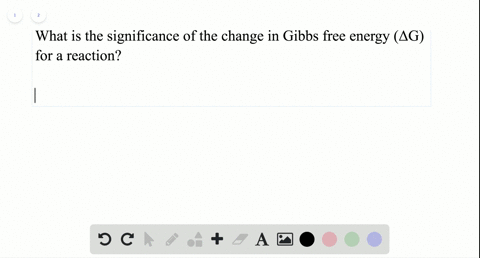### What is the significance of the change in Gibbs f…

01:06
LP
Problem 14

Why do exothermic processes tend to be spontaneous at low temperatures? Why does their tendency toward spontaneity decrease with increasing temperature?

Exothermic processes tend to be spontaneous at low temperatures because
the decrease in entropy of the system is overcome by a large increase in the
entropy of the surroundings, resulting in a positive $\Delta S_{u n i v} .$ Their tendency towards spontaneity decreases with increasing temperature, because the
magnitude of the positive $\Delta S_{\text {surr}}$ is smaller at higher temperatures.

## Discussion

You must be signed in to discuss.

## Video Transcript

So this question is asking why her ex a thermic reactions there are normally spontaneous at lower temperatures and as you increase temperature, tend to become non spontaneous. So we need to go back to what is one terms of reaction of spontaneous, non spontaneous. And for that we look at Delta G. So Delta G is positive. Reaction is known spontaneous, and Delta G is negative. Direction is spontaneous and for an exit, thermic reaction. Don't the H is negative because he is given up to surroundings. So no need to relate these two variables to each other. So w g equals, Does it h minus teed up the S. And so now you think about all right. If Delta H is always negative, then what would make Delta G negative? So temperature is always positive. So anything that can affect this is the scale of temperature and whether entropy is negative or positive venture be so there's two cases. Entropy is positive. Then this whole value is negative. In which case don't gs negative because you have a negative master negative and I'll give you a negative if don't s is negative. This value now becomes positive and now those edgy depends on the scale of temperature. Um so T is very big. I would just say be for big. The temperature is very large. Then this value will overcome your delta H value in Delta Julie Positive if entropy is still negative. But he is now pretty small now. Delta H. If temperatures small enough dealt H will overcome. However positive this number is in Delta G will be negative. And so that's why Rex anthemic reactions. They're typically spontaneous at lower temperatures, as you can see, because that lets your doctor GP negative.